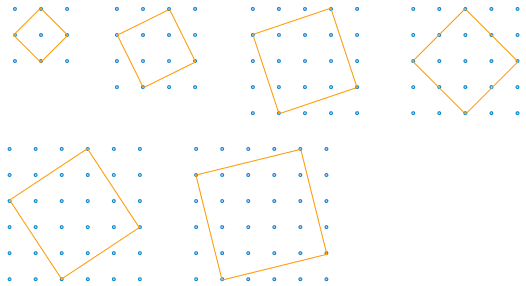# Problem 49582. Determine whether a square can be drawn on a grid of dots

Suppose you have a regular grid of dots, each 1 unit apart. By connecting four dots arranged in a square of side 1, you can draw a square of area 1. Similarly, you can draw squares of area 4, 9, 16, 25, etc. As shown below, by connecting dots with diagonal lines, you can draw squares of area 2, 5, 10, 8, 13, and 17. Which squares can you draw by connecting dots on the grid with straight lines?
Write a function that takes an area and determines whether the square of that area can be drawn.### Solution Stats

33.33% Correct | 66.67% Incorrect
Last Solution submitted on Jun 02, 2023

### Community Treasure Hunt

Find the treasures in MATLAB Central and discover how the community can help you!

Start Hunting!